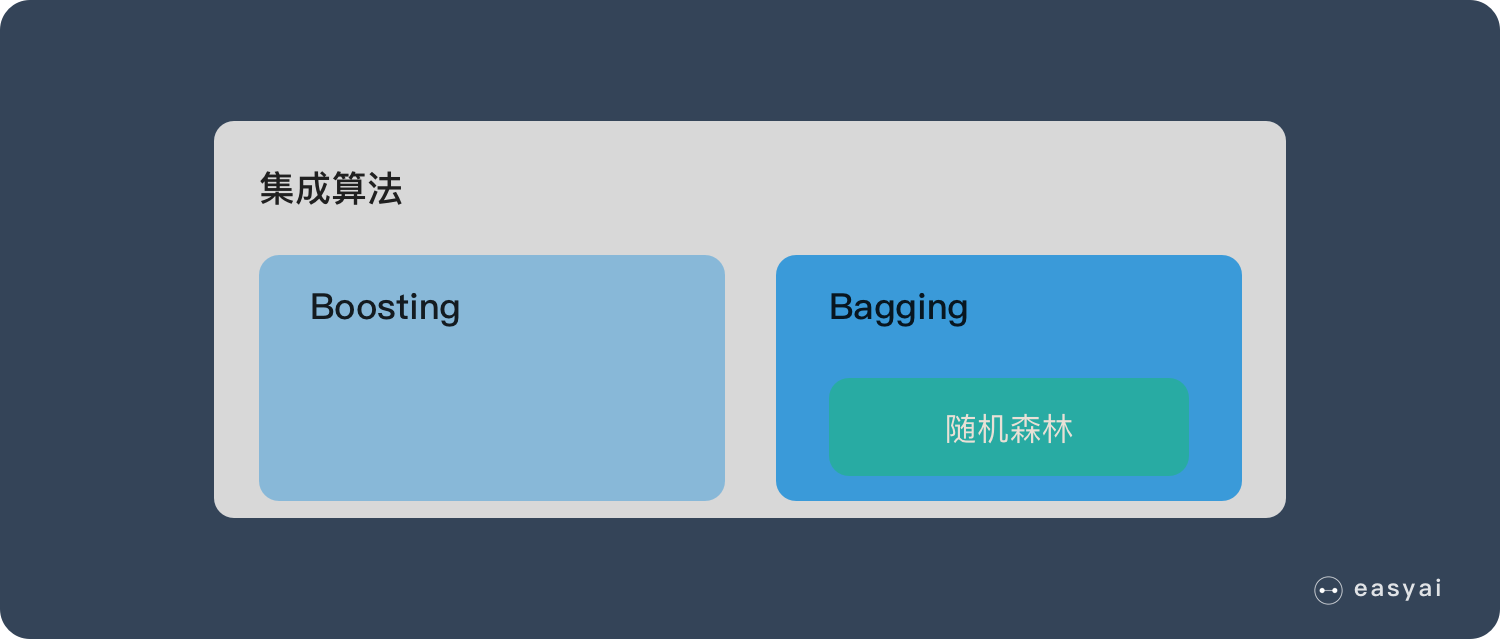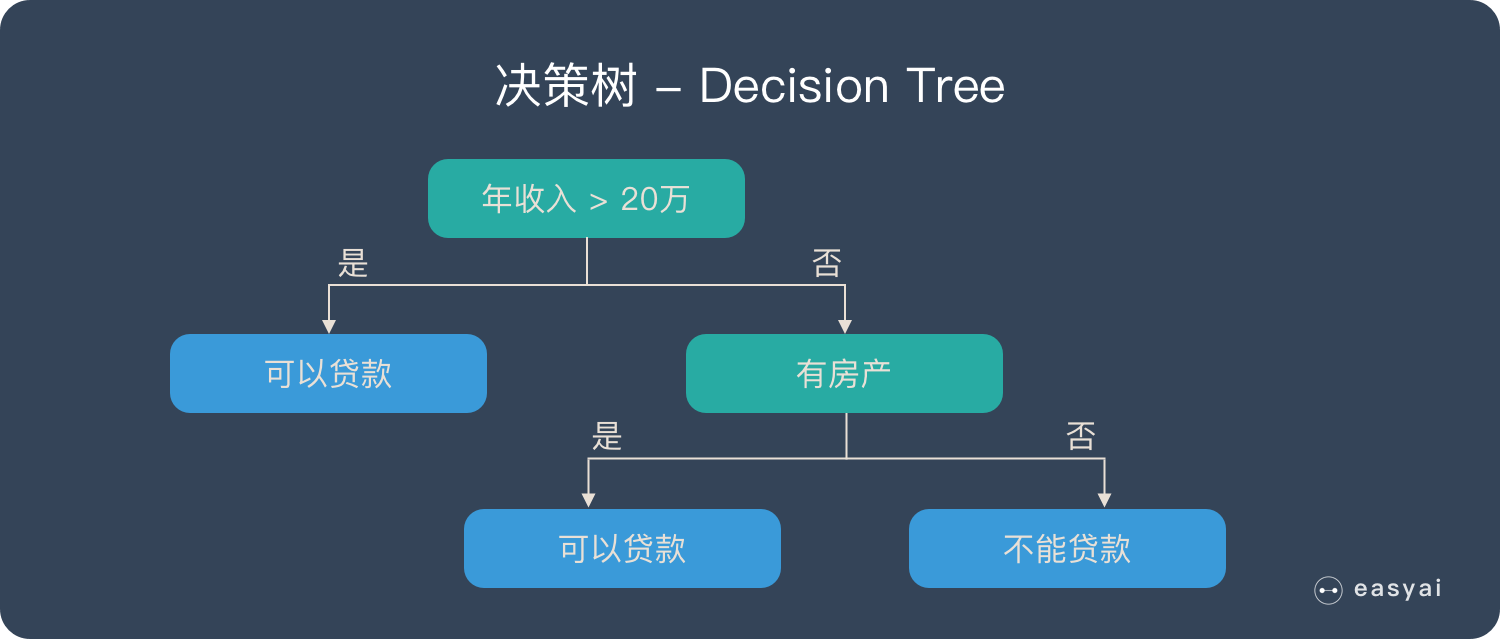11月 30, 2023

Processing Machinery

# Random Forest algorithm (Random Forest) Python implementation## foreword

Recently, I was studying a paper “Mining Quality Phrases from Massive Text Corpora”, which talked about how to mine high-quality phrases from massive text corpora, which used the Random Forest (Random Forest) algorithm, so I went to study it. Before I blogged It has been explained specifically for Decision Tree. Random Forest is an optimized version based on Decision Tree. Let’s discuss what Random Forest is.

## 1. What is Random Forest?

As a highly flexible machine learning algorithm, Random Forest (RF) has a wide range of application prospects, from marketing to health care insurance, it can be used for marketing simulation modeling, customer source statistics, retention and loss, can also be used to predict disease risk and susceptibility of patients. In domestic and foreign competitions in recent years, including the 2013 Baidu Campus Movie Recommendation System Competition, the 2014 Alibaba Tianchi Big Data Competition and the Kaggle Data Science Competition, participants used random forests in a very high proportion. So it can be seen that Random Forest still has a considerable advantage in terms of accuracy.

Having said so much, what kind of algorithm is random forest?

If readers have been exposed to decision trees (Decision Tree), then it will be easy to understand what is a random forest. Random forest is an algorithm that integrates multiple trees through the idea of ensemble learning. Its basic unit is a decision tree, and its essence belongs to a large branch of machine learning – the ensemble learning method. There are two keywords in the name of random forest, one is “random” and the other is “forest”. We understand “forest” very well. If one tree is called a tree, then hundreds or thousands of trees can be called a forest. This metaphor is very appropriate. In fact, this is also the main idea of random forest – the embodiment of integration thinking. The meaning of “random” will be discussed in the next section.

In fact, from an intuitive point of view, each decision tree is a classifier (assuming that it is now targeting a classification problem), then for an input sample, N trees will have N classification results. The random forest integrates all the classification voting results, and designates the category with the most votes as the final output. This is the simplest Bagging idea.Random Forest is a powerful and versatile supervised machine learning algorithm that grows and combines multiple decision trees to create a “forest”. It can be used for classification and regression problems in R and Python.

Before we explore random forests in more detail, let’s break it down:

What is supervised learning?
What are classification and regression?
What is a decision tree?
Understanding each of these concepts will help you understand random forests and how they work. So let’s explain.

1.1 What is supervised machine learning?

A function (model parameters) is learned from a given training data set, and when new data arrives, results can be predicted based on this function. The training set requirements for supervised learning include input and output, which can also be said to be features and targets. Objects in the training set are annotated by humans. Supervised learning is the most common classification (attention and clustering distinction) problem. An optimal model (this model belongs to a set of functions, The optimal means that it is the best under a certain evaluation criterion), and then use this model to map all the inputs to the corresponding outputs, and make simple judgments on the outputs to achieve the purpose of classification. It also has the ability to classify unknown data. The goal of supervised learning is often to let the computer learn the classification system (model) that we have created.

Supervised learning is a common technique for training neural networks and decision trees. These two techniques highly rely on the information given by the pre-determined classification system. For neural networks, the classification system uses the information to judge the error of the network, and then continuously adjusts the network parameters. With decision trees, classification systems use it to determine which attributes provide the most information. Typical examples in supervised learning are KNN and SVM.

1.2 What are regression and classification?

In machine learning, algorithms are used to classify certain observations, events, or inputs into groups. For example, a spam filter will classify every email as “spam” or “not spam.” However, the email example is just a simple example; in a business setting, the predictive power of these models can have a significant impact on how decisions are made and how strategies are formed, but more on that later.

So: both regression and classification are supervised machine learning problems that are used to predict an outcome or the value or class of an outcome. Their difference is:

Classification problems are used to put a label on things, usually the result is a discrete value. For example, to judge whether the animal in a picture is a cat or a dog, classification is usually based on regression, and the last layer of classification usually uses the softmax function to judge its category. Classification does not have the concept of approximation, and there is only one correct result in the end, and the wrong one is wrong, and there will be no similar concept. The most common classification method is logistic regression, or logistic classification.

Regression problems are usually used to predict a value, such as predicting housing prices, future weather conditions, etc. For example, the actual price of a product is 500 yuan, and the predicted value through regression analysis is 499 yuan. We think this is a relatively good regression analysis . A more common regression algorithm is the linear regression algorithm (LR). In addition, regression analysis is used on the neural network, and the top layer does not need to add the softmax function, but directly accumulates the previous layer. Regression is an approximate prediction of the true value.

A simple way to distinguish between the two can be roughly stated that classification is about predicting labels (such as “spam” or “not spam”), while regression is about predicting quantities.

1.3 What is a decision tree?

Before explaining random forests, we need to mention decision trees. Decision tree is a very simple algorithm, which is highly explanatory and conforms to human intuitive thinking. This is a supervised learning algorithm based on if-then-else rules. The above picture can intuitively express the logic of the decision tree.The derivation process of the decision tree is described in detail in my previous blog

Machine Learning – Decision Tree (1)

Machine Learning – Derivation of Decision Trees

1.4 What is Random Forest?
A random forest is composed of many decision trees, and there is no correlation between different decision trees.

When we perform a classification task, when a new input sample enters, each decision tree in the forest is judged and classified separately. Each decision tree will get its own classification result, which classification in the classification result of the decision tree At most, then Random Forest will take this result as the final result.
———————————————